# TANGENT AND NORMAL Solutions, Inter first year mathematics 1B problems with solutions

Mathematics Intermediate1B solutions for some problems for Tangents and Normal.

These solutions are very simple to understand.

Study the lesson Applications of Derivatives very well.

Observe the example problems and solutions given in the textbook.

Applications of Derivatives

Exercise 10(a)

Exercise 10(b)

Exercise 10(c)

Exercise 10(d)

Exercise 10(e)

Exercise 10(f)

Exercise 10(g)

Exercise 10(h)

You can also see

Inter Maths 1A textbook solutions

Inter Maths 1B textbook solutions

Inter Maths IIA textbook solutions

Inter Maths IIB textbook solutions

M

## Calculus, Tangent and Normal Junior Inter Maths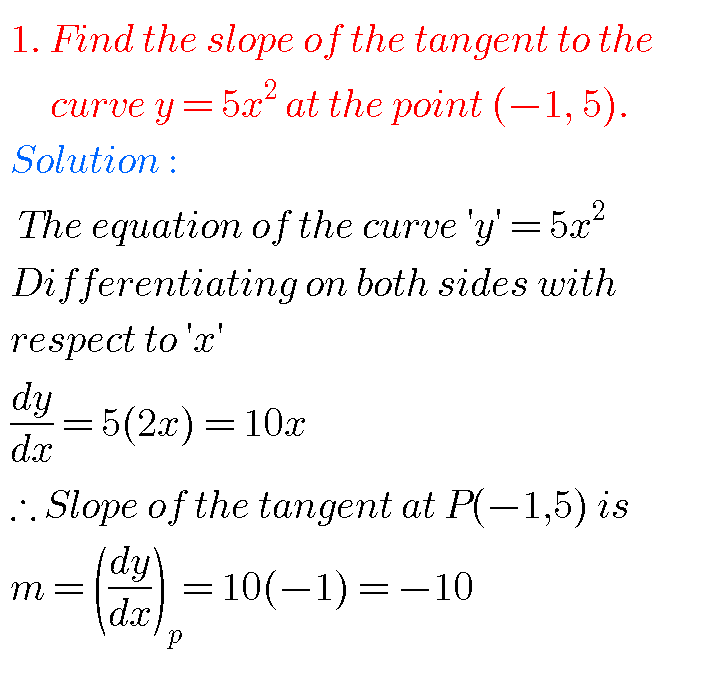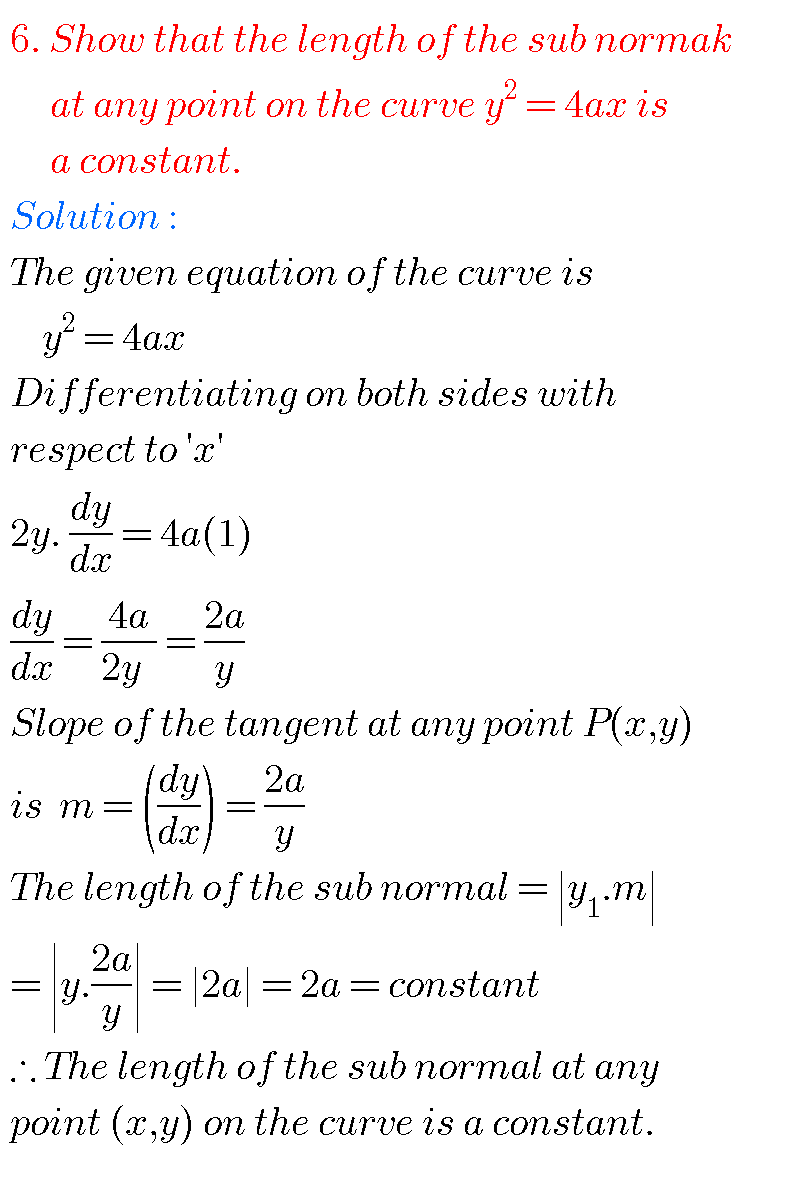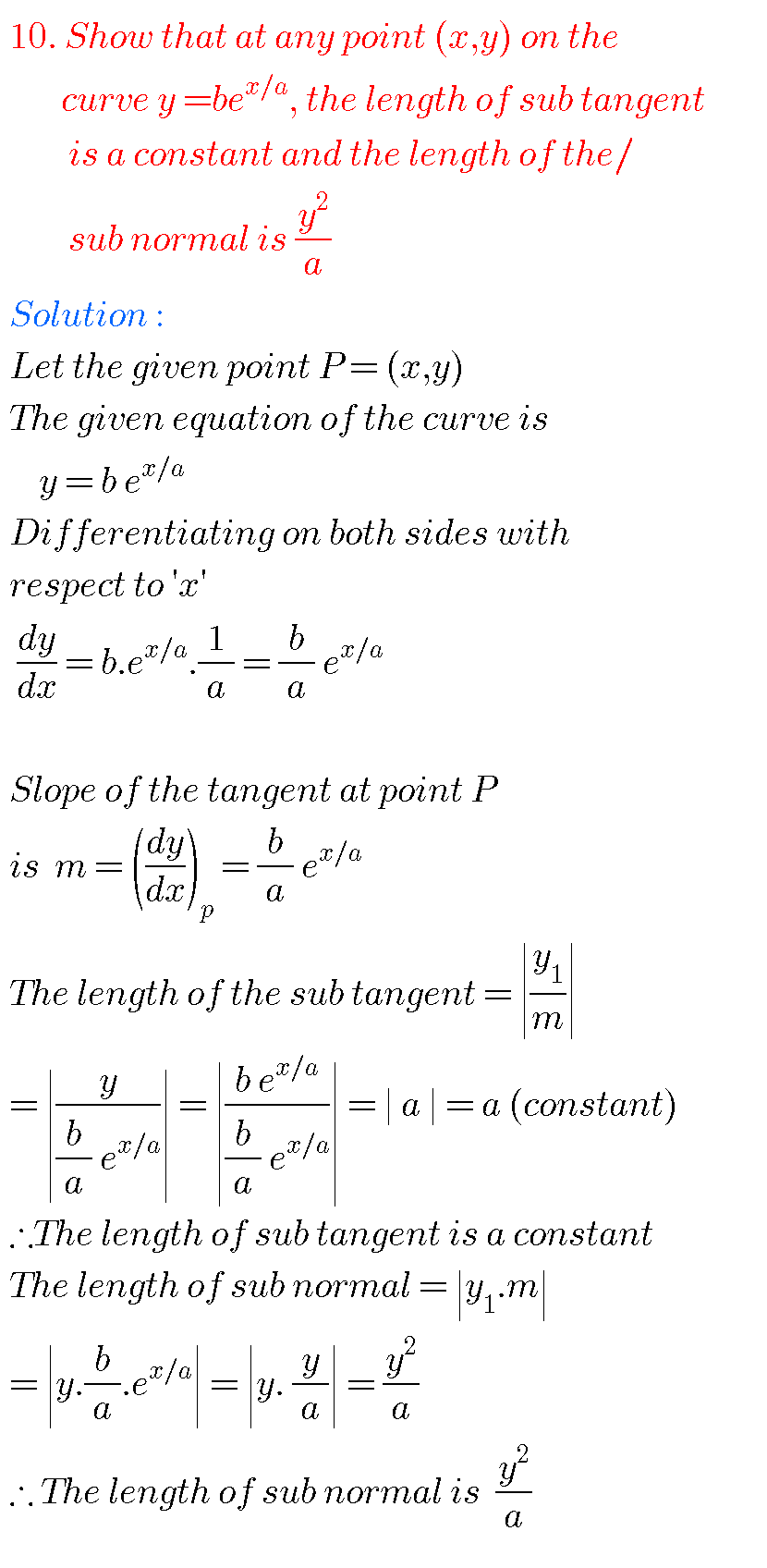Problem 11## Inter maths tangent and normal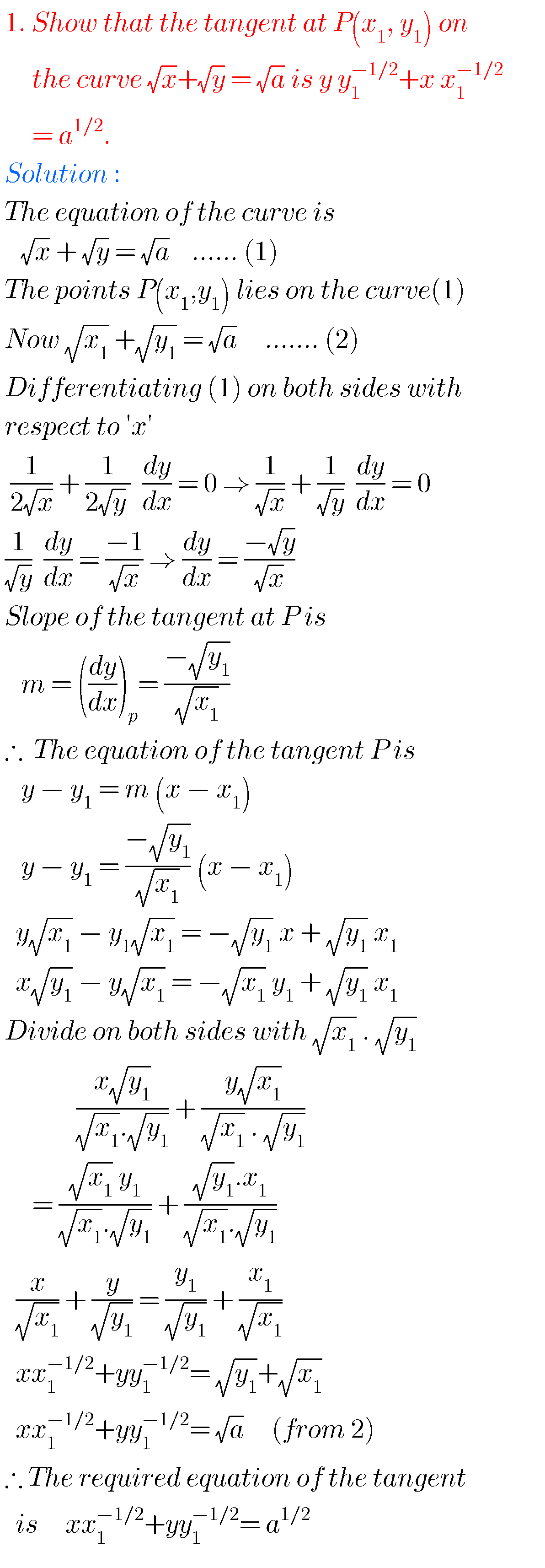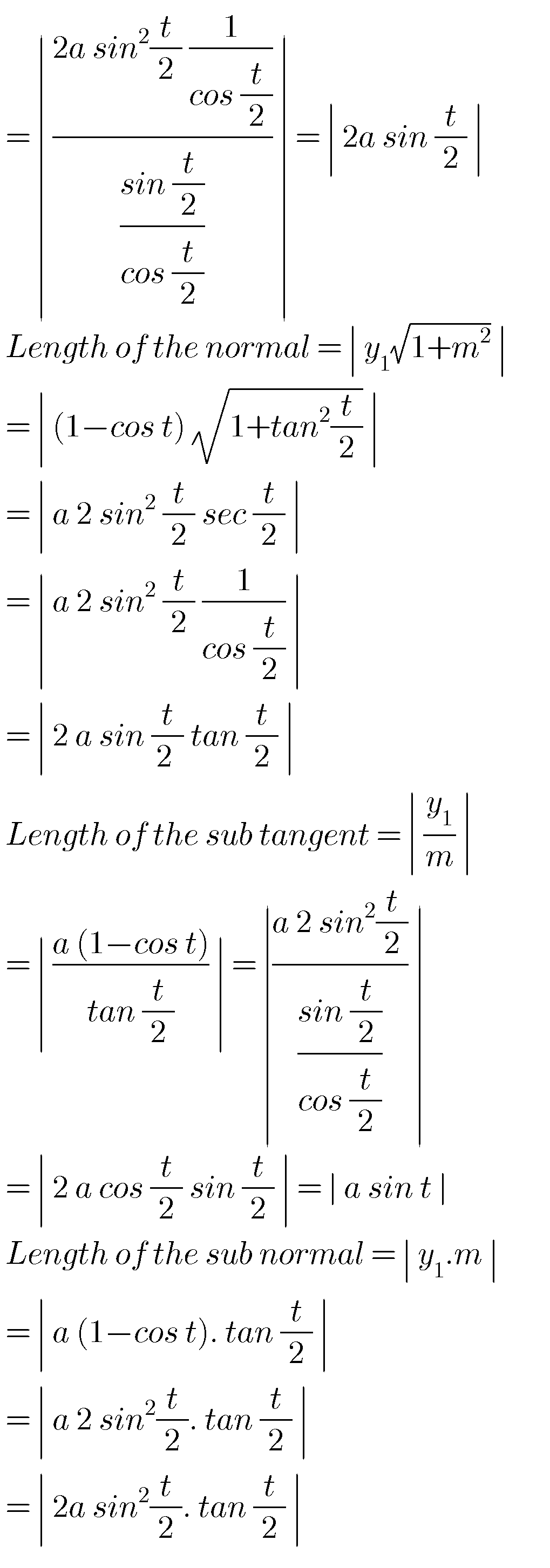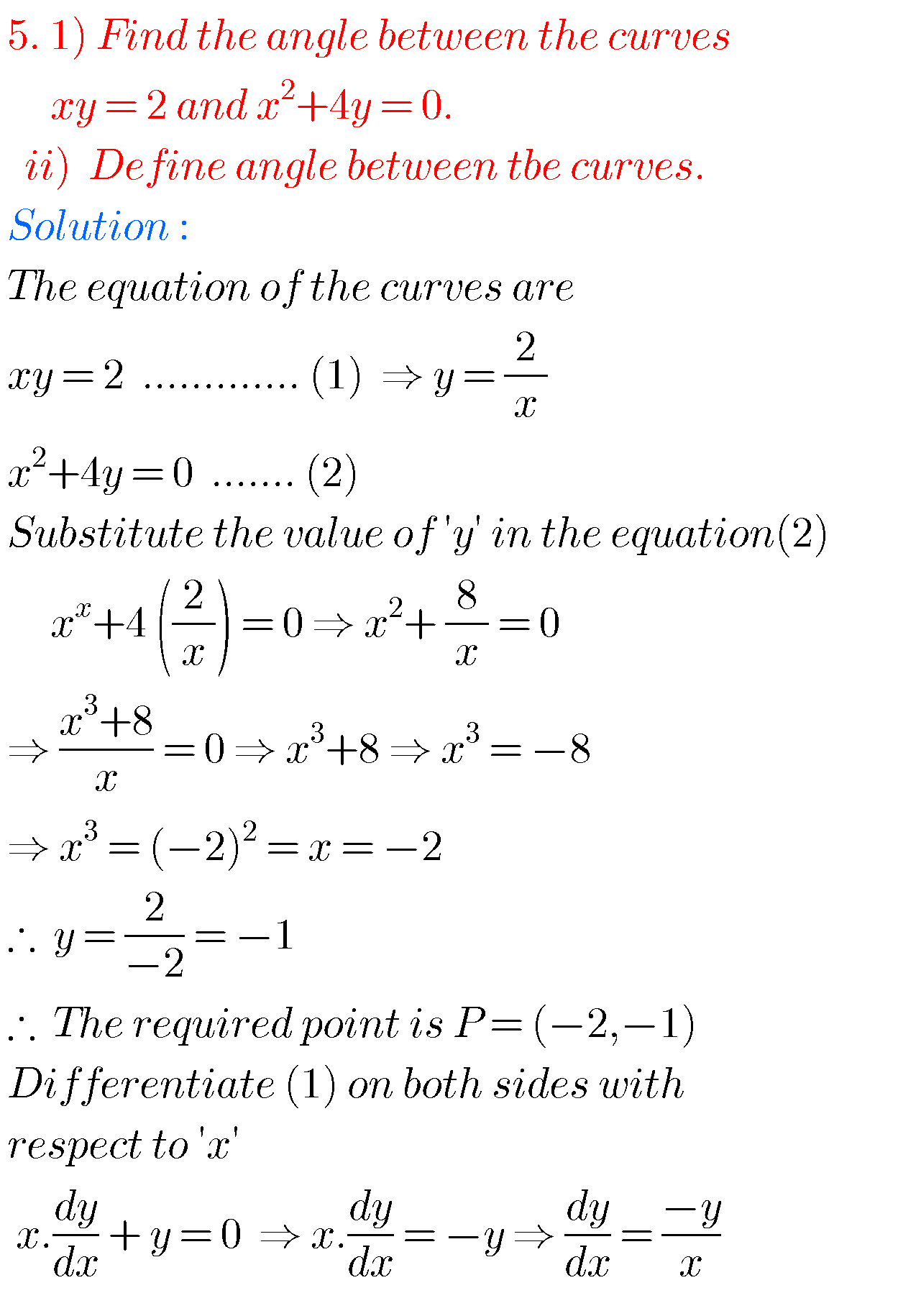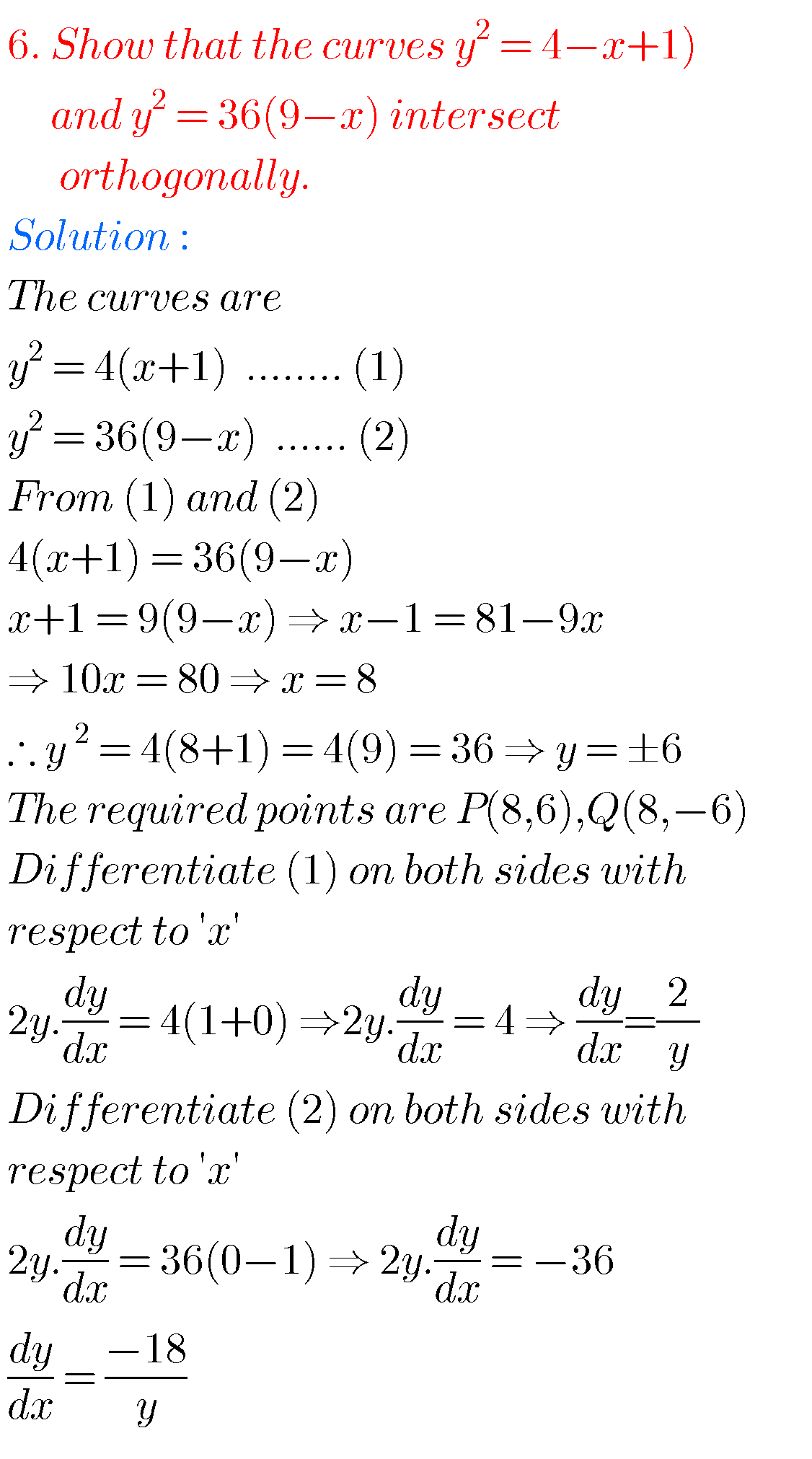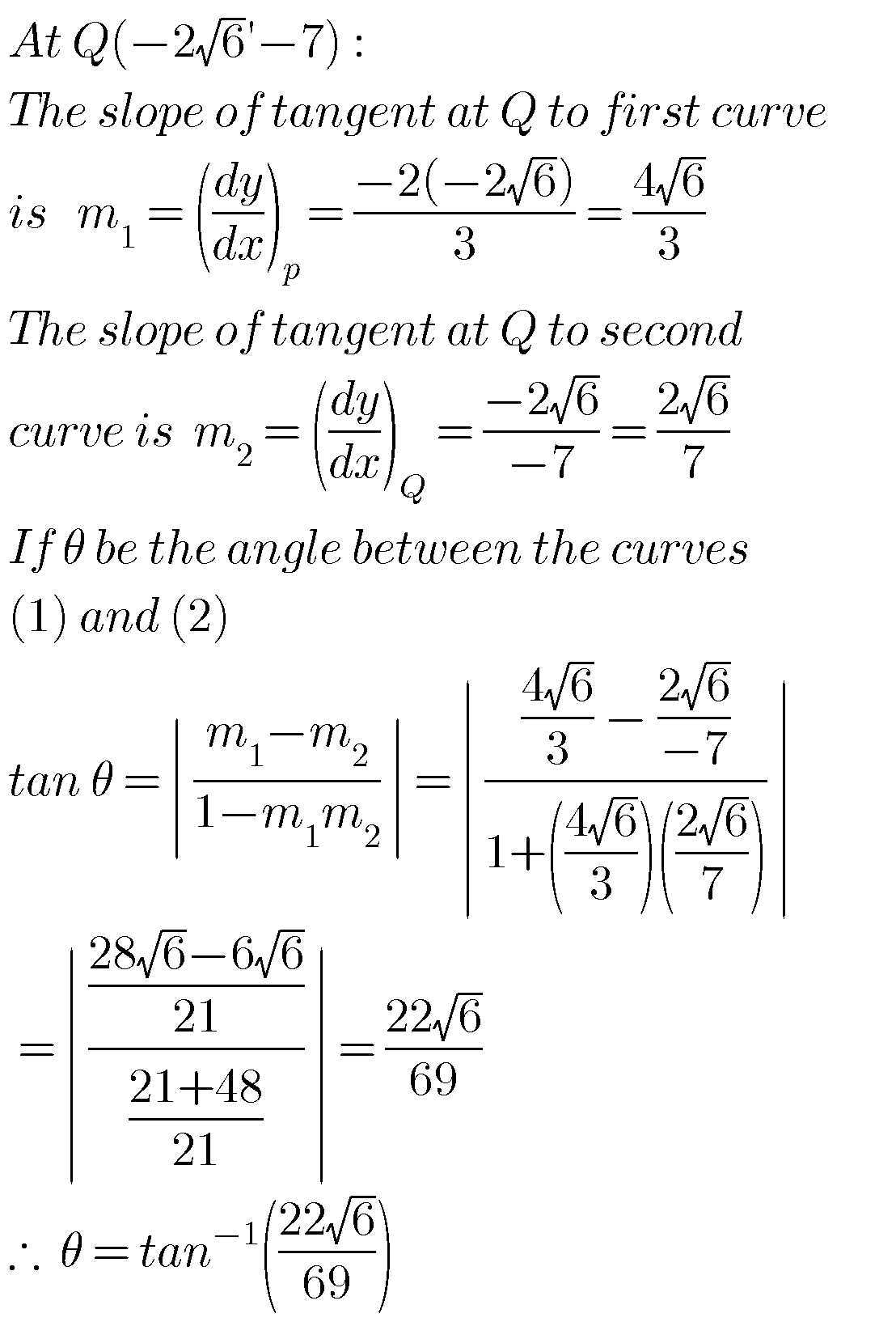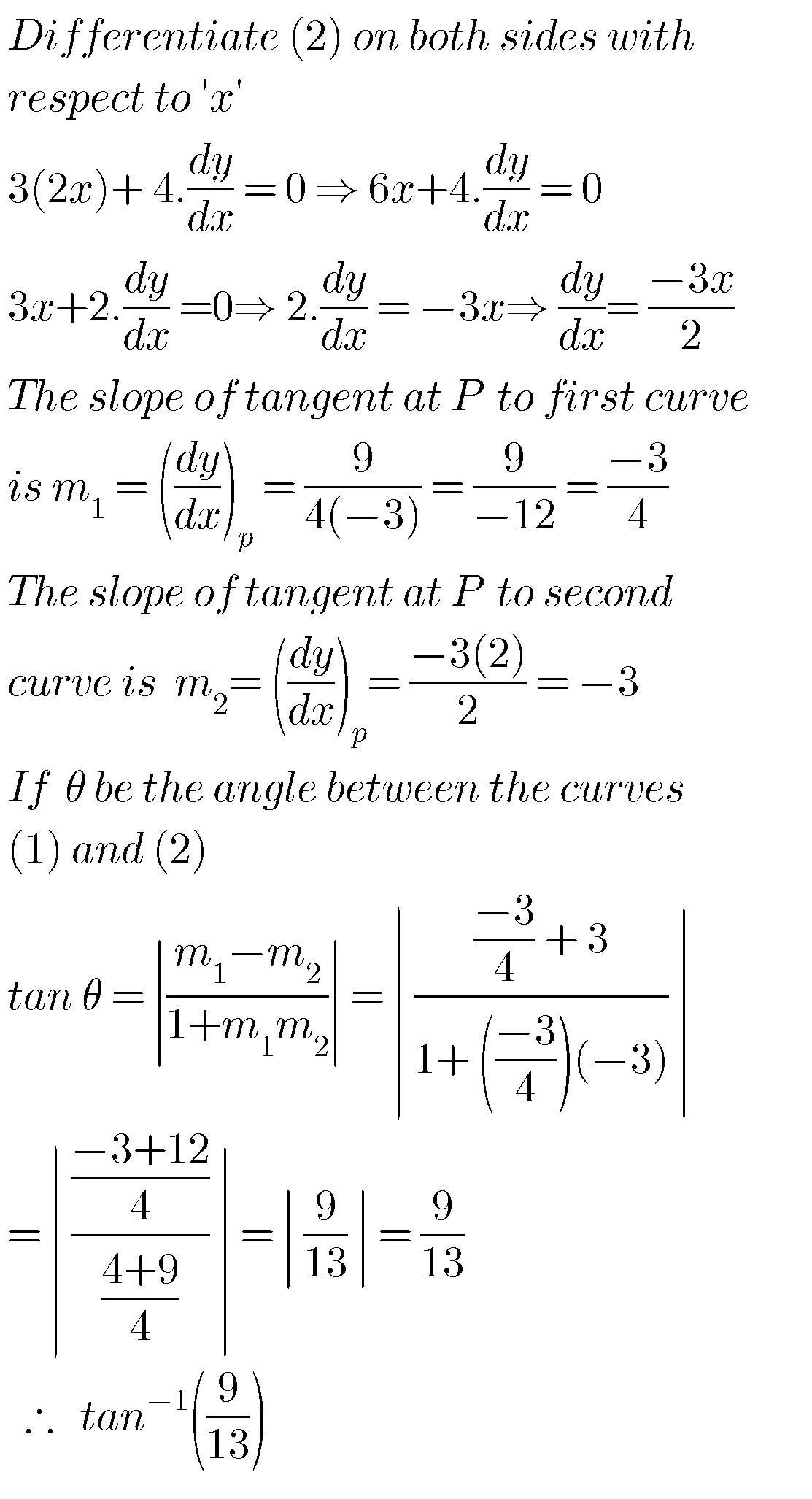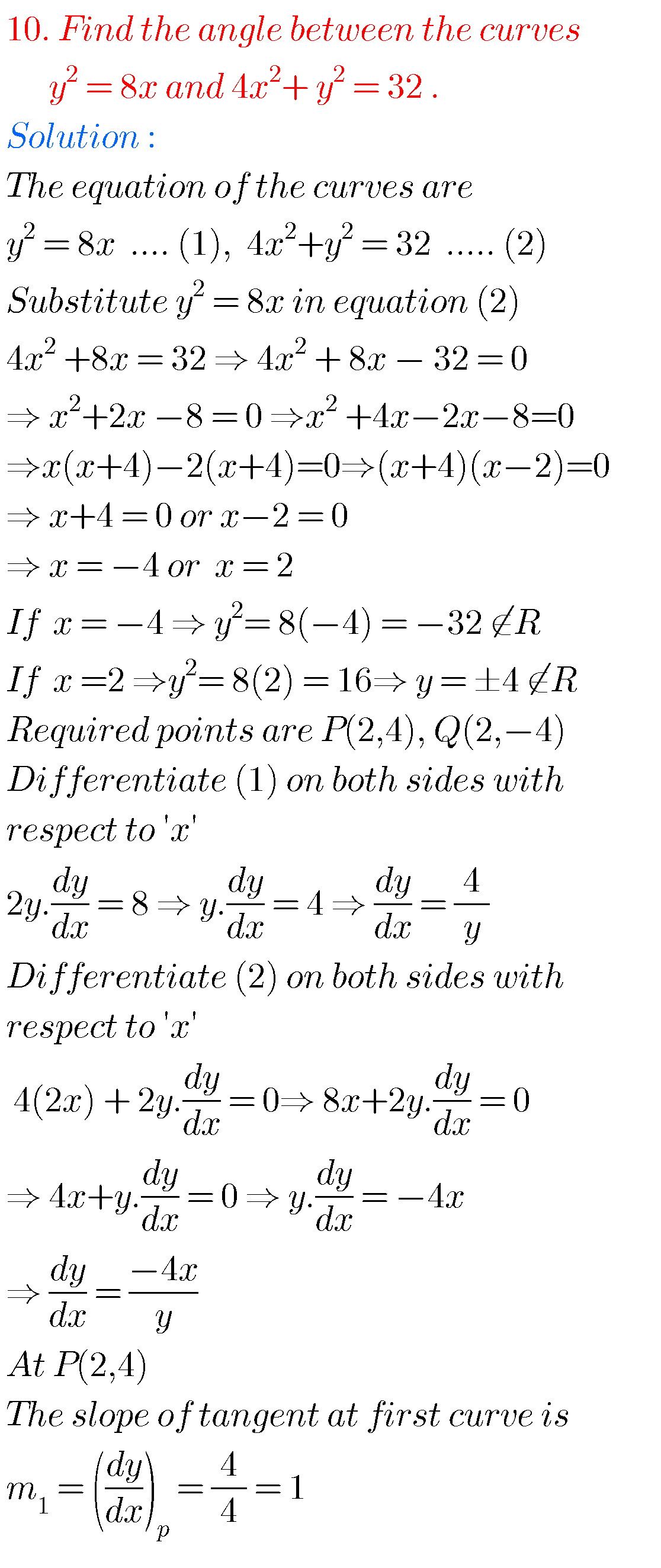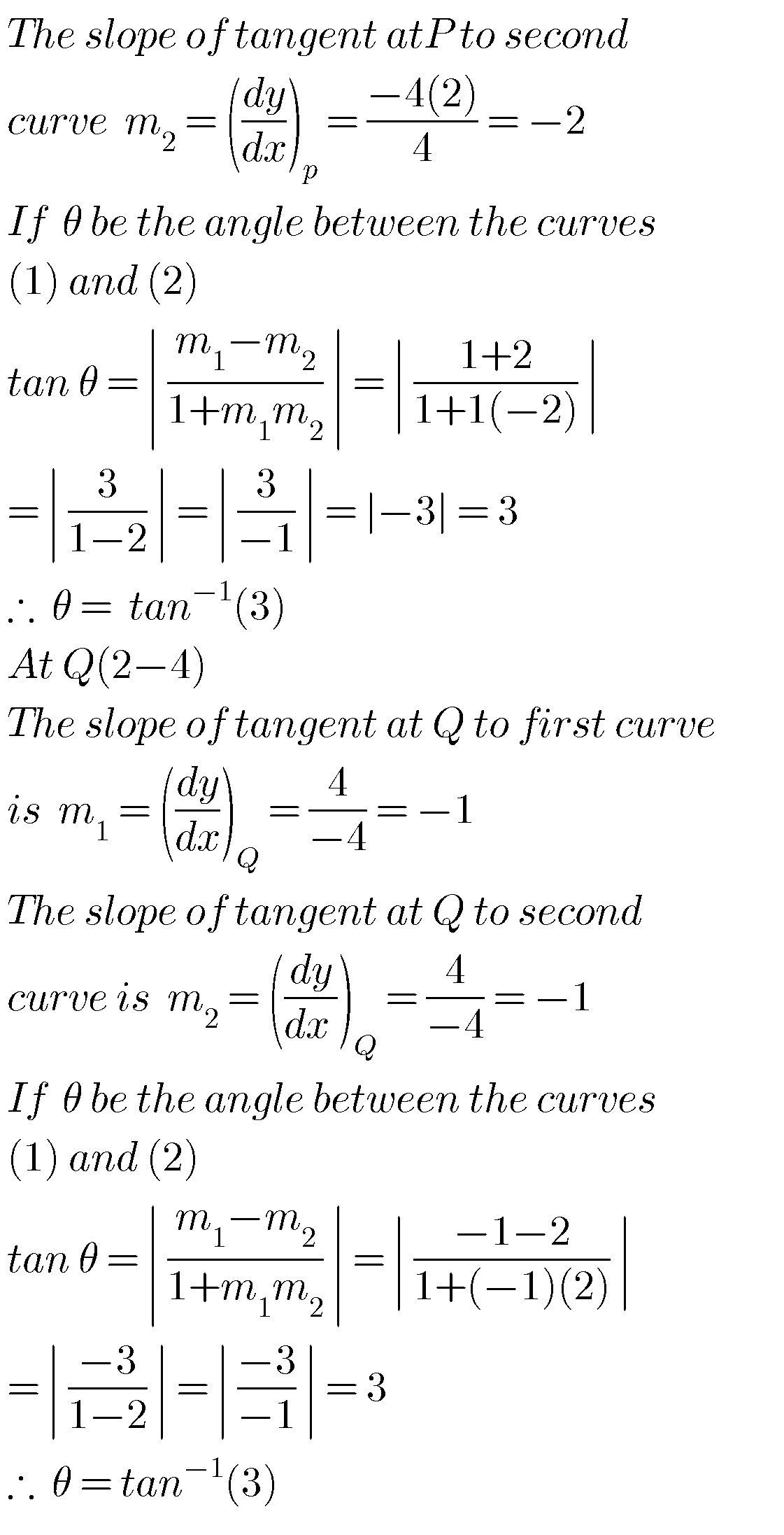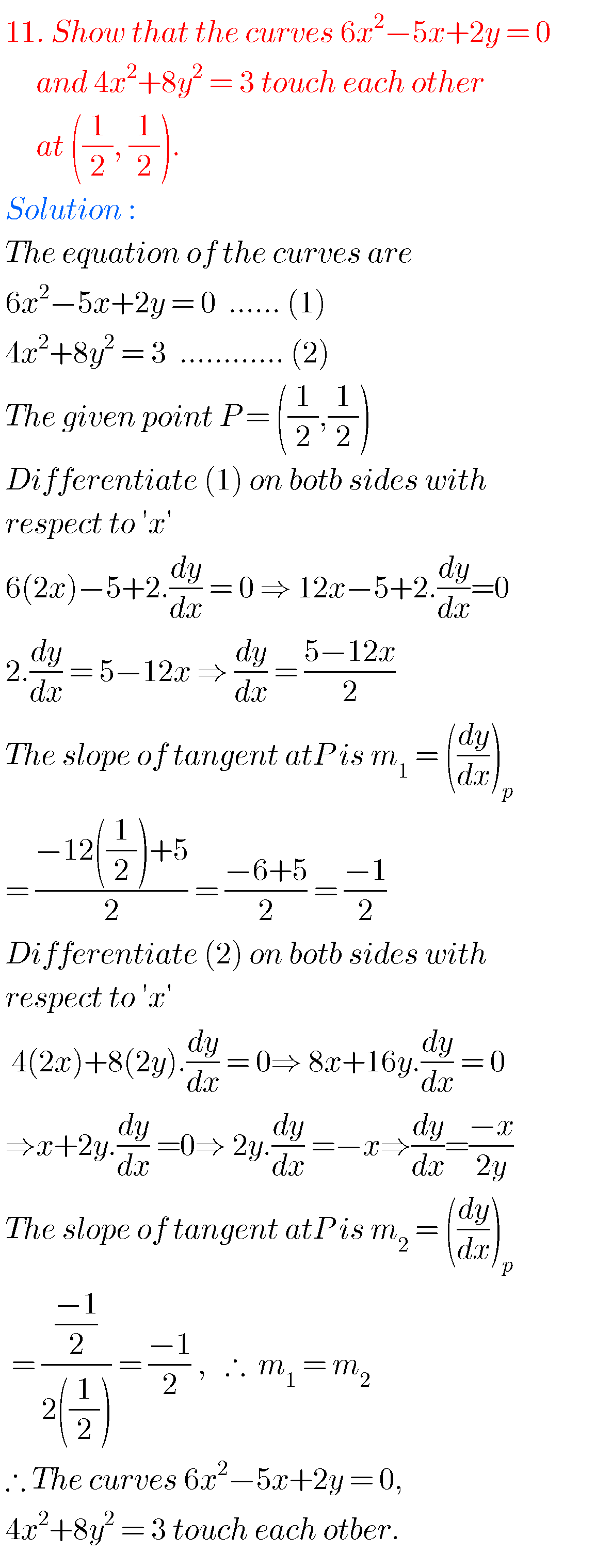Note : Observe the solutions and try them in your own methods.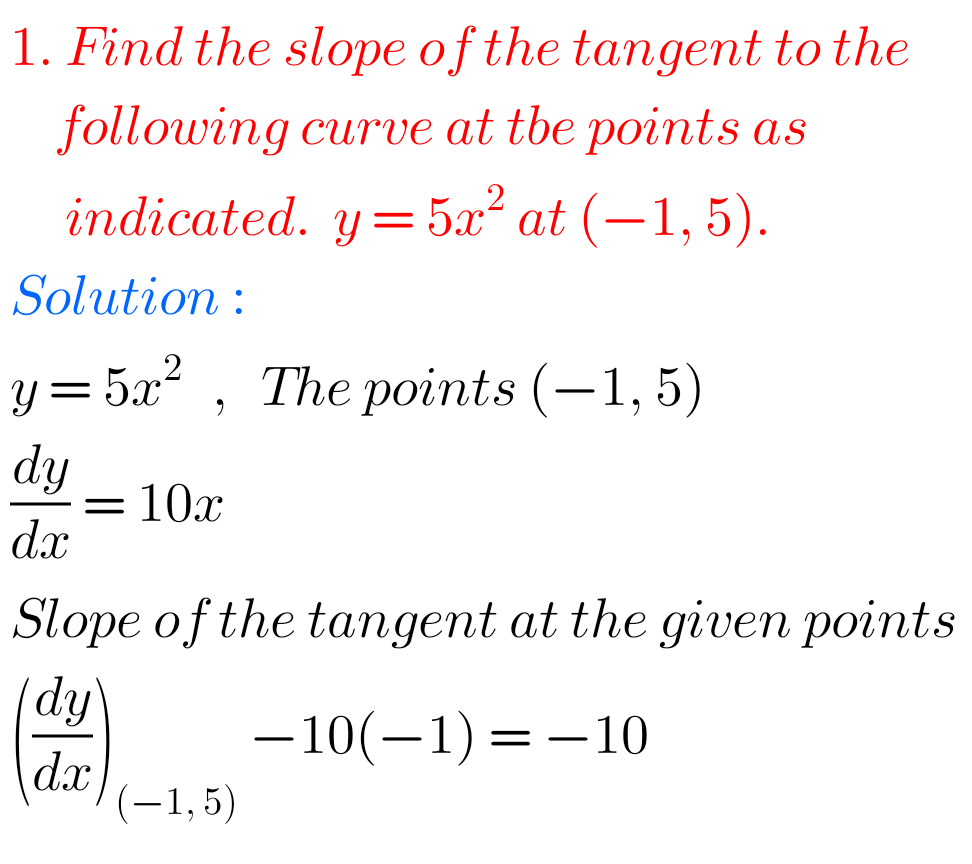You can see the solutions for junior inter maths 1B

1. Locus

The straight line sa

Straight lines la

11. Rate measure

Maths real numbers solutions class 10